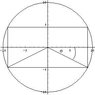# Word Problem

powp
Hello All

I have this word problem that I am having problems with

Here it is

A circular piece of sheet metal has a diameter of 20 in. The edges are to be cut off to form a rectangle of area in^2. What are dimensions of the rectangle??

Here is what I have figured out. Not sure if it of any use

Formula of Circle is

10^2 = y ^ 2 + x ^ 2

Area of rectangle

160 = LW

Thanks

Homework Helper
powp said:
A circular piece of sheet metal has a diameter of 20 in. The edges are to be cut off to form a rectangle of area in^2. What are dimensions of the rectangle??

Here is what I have figured out. Not sure if it of any use

Formula of Circle is

10^2 = y ^ 2 + x ^ 2

Area of rectangle

160 = LW

Thanks

So, as I see it you have a circle of radius 10 and want to draw a rectangle in it with a total area of 160 right? Look at the plot below (drawn to scale) and where I have theta. Can you figure out what the length and height has to be using some trig expressions?

#### Attachments

•recin circle.JPG
8.4 KB · Views: 376
powp
Don't think I have enough info to use trig expessions. All I know for that triangle is the hypotenuse is 10 in and is a right angle triangle.

How can I determine the remaining info?
if I use sin I need opposite side?

plucker_08
IT’S ALL GEOMETRY…

X^2 + Y^2 = 20^2 = 400 ----------------------DIAGONAL OF THE RECTANGLE

XY = 160
2XY = 320

X^2 + 2XY + Y^2 = 400 + 320 = 720
(X+Y) ^2= 720

X+Y = 12 √5

X^2 – 2XY + Y^2 = 400 – 320 = 80
(X-Y)^ 2 = 80

X-Y = 4 √5

2 EQS., 2 UNKNOWNS

2X = 4 √5 + 12 √5 = 16 √5

X = 8 √5
Y = 4√5

MaxwellPhill
Nice explanation plucker.

powp
thanks why did you multiply xy = 160 by 2 ??

plucker_08
adding 2xy to x^2 + y^2 will yield to an expression x^2 + 2xy + y^2 which is the square of the sum of the sides of the rectangle...(x + y)

subtracting 2xy to x^2 + y^2 will yield to an expression x^2 - 2xy + y^2 which is the square of the difference of the sides of the rectangle...(x - y)

you will have 2 eqs and 2 unknows...

powp
how can you just add values to the equation?? How do you know when to do this?

Homework Helper
Hey Powp. Sorry for not get getting back with you last night but looks like these guys helped you ok. Yea, I didnt' see the obvious algegra:

$$x^2+y^2=400$$

$$xy=160$$

Solving for x or y in the second equation and substituting into the first leads to the same equation for both x and y:

$$r^4-400r^2+160^2=0$$

Now, using the quadratic formula, just figure out which of the 4 roots make sense.

I drew a pretty graph though.Last edited: